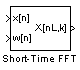# Short-Time FFT

Nonparametric estimate of spectrum using short-time fast Fourier transform (STFT) method

• Library:
• DSP System Toolbox / Transforms

•## Description

The Short-Time FFT block computes a nonparametric estimate of the spectrum. The block buffers, applies a window, and zero pads the input signal. The block then takes the FFT of the signal, transforming it into the frequency domain.

## Ports

### Input

expand all

Specify the time-domain input signal as a vector or a matrix. If the input is a matrix, the block treats each column as an independent channel. The input can be a variable-size signal. That is, the frame size of the signal can change while the simulation is running. The number of channels must remain the same.

If the input is fixed point, it must be a signed integer or signed fixed point with a power-of-two slope and zero bias.

Data Types: `single` | `double` | `int8` | `int16` | `int32` | `fixed point`
Complex Number Support: Yes

Connect your single-channel analysis window to the w[n] port. For the Analysis window length parameter, enter the length of the analysis window W. The block buffers the input signal such that it has a frame length of W.

If the window vector is fixed point, it must be a signed integer or signed fixed point with a power-of-two slope and zero bias.

Data Types: `single` | `double` | `int8` | `int16` | `int32` | `fixed point`

### Output

expand all

The block outputs the complex-valued single-channel or multichannel short-time FFT at this port.

Data Types: `single` | `double` | `int8` | `int16` | `int32` | `fixed point`
Complex Number Support: Yes

## Parameters

expand all

Specify the frame length of the analysis window as a positive integer greater than 1. The block buffers the input into overlapping window segments using the specified window length and overlap length, and then multiplies each overlapped segment by the window.

Specify the number of samples by which consecutive windows overlap in each frame of the input signal as a positive integer. The window overlap reduces artifacts at the data boundaries.

Enter the length up to which the block pads the input signal as a positive integer. After the block buffers and windows the signal, it zero-pads the signal before computing the FFT. This parameter determines the length of the short-time fast Fourier transform output (number of rows). The FFT length must be greater than or equal to the window length.

## Block Characteristics

 Data Types `double` | `fixed point` | `integer` | `single` Direct Feedthrough `no` Multidimensional Signals `no` Variable-Size Signals `no` Zero-Crossing Detection `no`